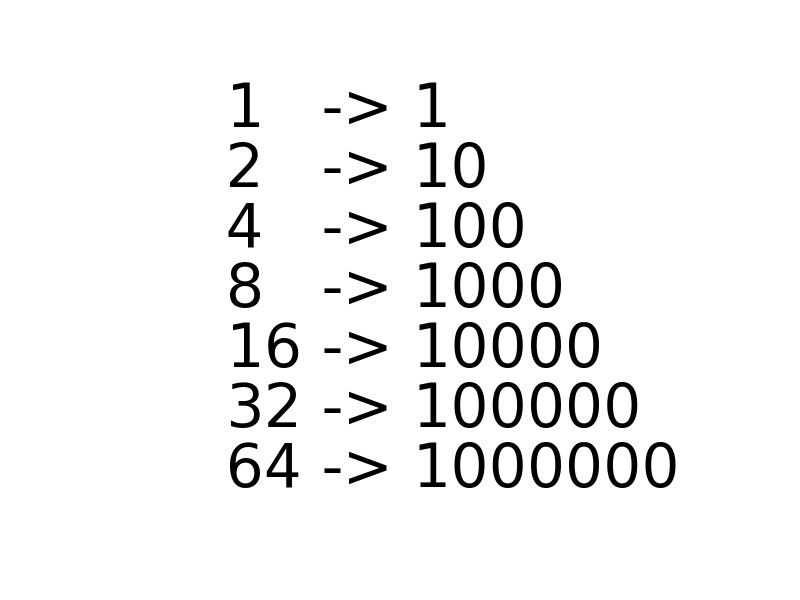×

Search anything:

# Detect if a number is power of 2 using bitwise operators

#### bitwise operation and operation power of 2 List of Mathematical AlgorithmsGet this book -> Problems on Array: For Interviews and Competitive Programming

Reading time: 15 minutes | Coding time: 1 minutes

Bitwise operators are one of the most under-rated operators in C/C++, despite being quite fast as most of the processors today have direct support for bit level operators.

In this article, we will explore how to determine if a number is a power of 2 based on bitwise operations.

### Algorithm

We need to check if a number is power of 2 or not.

An obvious and trivial solution which comes to mind is keep dividing the number by 2 until you cannot and check if the number is 1 or 0.

On thinking further, we see that we are taking O(log(n)) to solve such a simple problem.

Can this be improved?

Of course, and Bitwise operators come to our rescue. But before that we need to study simple property of binary number which are powers of 2.Can you see where we are going?

The powers of 2 have only one set bit in their Binary representation.

Let me be more explicit. If we subtract 1 from a power of 2 what we get is 1s till the last unset bit and if we apply Bitwise AND operator we should get only zeros.

This whole algorithm has a complexity of O(1).

We can prove the correctness of algorithm.

Suppose if there is a 1 to the left of first set bit, i.e., it is not a power of 2. We can clearly see that `n & (n - 1)` will not return 0.

16 0 0 0 1 0 0 0 0
16-1=15 0 0 0 0 1 1 1 1
16 AND 15 0 0 0 0 0 0 0 0

Hence, 16 is a power of 2.

15 0 0 0 0 1 1 1 1
15-1=14 0 0 0 0 1 1 1 0
15 AND 14 0 0 0 0 1 1 1 0

Hence, 15 is not a power of 2.

• C++

### C++

``````
#include <iostream>
// Function to check if the number "x" is power of 4
bool is_power_of_2(int x)
{
// Check if the number has only one set bit
if ((x & (x - 1)) != 0)
return false;
return true;
}
int main(int argc, char *argv[])
{
int n;
std::cout << "Enter a number:" << std::endl;
std::cin >> n;
// Print the result
std::cout << ((is_power_of_2(n)) ? "YES" : "NO") << std::endl;
return 0;
}
``````

### Complexity

• Worst case time complexity: `Θ(1)`
• Average case time complexity: `Θ(1)`
• Best case time complexity: `Θ(1)`
• Space complexity: `Θ(1)`

### Questions

#### The algorithm works for:

Positive integers
Integers
Negative integers
Real numbers#### OpenGenus Tech Review Team

The official account of OpenGenus's Technical Review Team. This team review all technical articles and incorporates peer feedback. The team consist of experts in the leading domains of Computing.

Improved & Reviewed by:

Detect if a number is power of 2 using bitwise operators